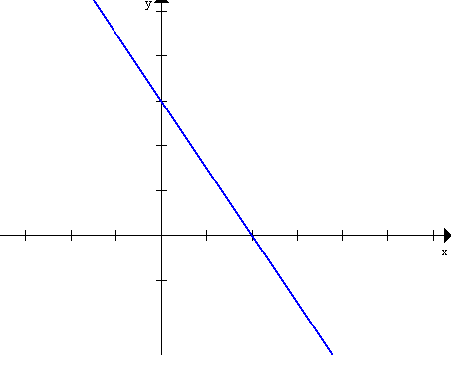Tuesday, January 20
First Class!
• Discussion of course details:
• What is Visual Mathematics?
• Mathematics that studies topics related to visual experience. [Geometry, Topology]
• Visualization of mathematics that is not inherently visual.
• Example: Numbers...
• numeral: a symbol for representing a number
• Number: a form of universal language to describe anything/ physical things/ concepts related to measurement

• such as V, 5, five, cinq, chamesh, cinco
• Frege distinguished numerals from numbers in the late 19th century.
• We can compare numbers... for instance we say" 3 is less than 5"
• Is 3 smaller than 5?
• Numerals are symbols (visual or linguistic) that we use to represent numbers.
• We use numbers to measure (lengths) and put things in order (which was first).
• Another common visual representaion of numbers uses the number line.
• ___.___.___.___.___.___.___.______
•       1     2     3     4     5     6     7

• Here there numerals are connected to points, so the points are considered to visualize the corresponding numbers.

• We visualize equations that  give relations between numbers with graphs in the coordinate plane.

• 3x + 2y = 6 is visualized by the graph of a line ...